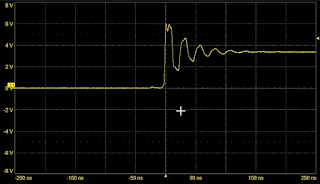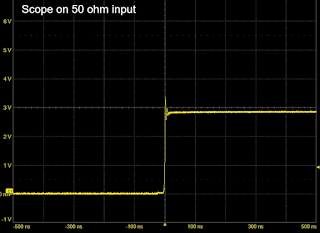## You need to test, we're here to help.

You need to test, we're here to help.

## 14 February 2022

### Transmission Lines for Oscilloscope Users, Part 4Figure 1: Characteristic waveform when the source impedance is lower than the cable impedance.
In Part 3, we saw the pattern of reflections that occur when both the source impedance and the oscilloscope input impedance are higher than that of the interconnect, and how those reflections affected the rise time measurement. Now let’s briefly consider what happens when the source impedance is lower than the impedance of the connecting cable.

For this example, the source voltage is a 3.3 V square wave and the source impedance is 9 Ω. As before, our transmission line is a 50 Ω coaxial cable connecting the source to the oscilloscope. If the oscilloscope input termination is set to 1 MΩ, we see the interesting waveform shown in Figure 1.

The signal exhibits ringing on the leading edge. As in the previous example, this apparent ringing is due to reflections that are an artifact of the measurement system; it is not the ringing actually present in the source signal we are trying to measure.

Let’s use what we've learned about transmission lines to explain what we observe. The square wave from the ideal voltage source passes through the output impedance of 9 Ω and sees the 50 Ω impedance of the cable. This forms a voltage divider with a loss of about 15%, dropping the voltage swing to 2.8 V at the cable input.

The 2.8 V signal propagates down the cable until it hits the 1 MΩ oscilloscope input impedance, a virtual open circuit, where, as we saw before, it is 100% reflected.  The oscilloscope sees the sum of the 2.8 V incident and 2.8 V reflected signals, and measures a peak amplitude of 5.6 V.  The reflection travels back toward the source where it sees the 9 Ω source impedance.  Since the source impedance is lower than the cable impedance, the reflection is negative, with a reflection coefficient of 0.7. The signal is again reflected toward the oscilloscope, resulting in a valley before it again reflects toward the source. The oscilloscope records the reduced amplitude of approximately 2 V. This process of alternating positive and negative reflections continues until the reflection amplitude is reduced to zero and the voltage settles to the open circuit voltage of 3.3 V. The alternating peaks are not oscillatory ringing but are simply due to bipolar reflections.Figure 2: A 50 Ω termination eliminates reflections and the observed ringing is attributable to the source.
As we learned from the example in Part 3, when using 50 Ω coaxial cable, the cure for reflections is to terminate the measurement into 50 Ω. In Figure 2, the ringing seen on the signal is actually from the source and not an artifact of the measurement system.

Keep in mind that using the oscilloscope’s 50 Ω input may place an unacceptable load on the signal source. In such a case, an active probe should be used instead of a 50 Ω coaxial cable.

So, how does knowing this help us think differently about our measurements? Well, we have provided a framework for characterizing all the elements of our measurement circuit. Using the known input impedances of the oscilloscope and the characteristic impedance of the transmission line, we can fully characterize the Thevenin source signal. With this knowledge in hand, we can assess the potential for reflections and know how they may affect the measurement.

Watch Dr. Eric Bogatin explain these basic principles of signal integrity in our on-demand webinar, What Every Oscilloscope User Needs To know About Transmission Lines.

Ready to go beyond “Transmission Lines 101”? You’ll find “Transmission Lines 201” in our earlier series:

Transmission Lines, Part I

Transmission Lines, Part II